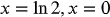1
63
views
50b
Problem

Problem 50b

Chapter 1: Functions and ModelsTextbook ExpertVerified Tutor
19 Oct 2021

Given information

The given equation is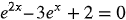.

Step-by-step explanation

Step 1.

Solve for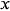given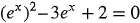rewrite the equation using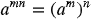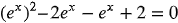expand the middle term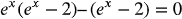write out common elements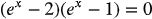write out common terms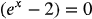or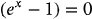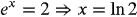or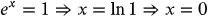Hence,